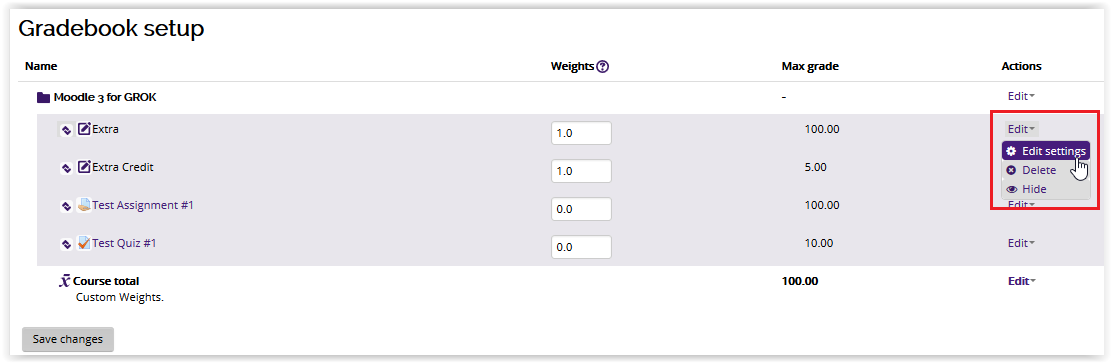#### General Information:

Moodle allows users to curve graded assignments using the Multiplicator tool. The Multiplicator is a factor by which all grades for a graded item will be multiplied, with a maximum value of the maximum grade.

For example, if the multiplicator is 2.0 and the maximum grade is 100, then all grades less than 50 are doubled (e.g. 35 => 70, 40 => 80, 45 => 90, etc.), and all grades 50 and above are changed to 100 (e.g. 55 => 100, 75 => 100, 85 => 100, etc.).

#### To Use the Grade Multiplicator Tool:3. Select Edit from under the Actions column, and Edit settings for the graded item you wish to edit.6. Type a value for the multiplicator.

• For Multiplicator values greater than 1.0, grades will increase
• For Multiplicator values equal to 1.0, grades will remain the same
• For Multiplicator values less than 1.0, grades will decrease.7. When you're finished, click Save Changes.Reference from: docs.moodle.org

18971
7/1/2020 2:47:44 PM